# AES算法简介

1、总体结构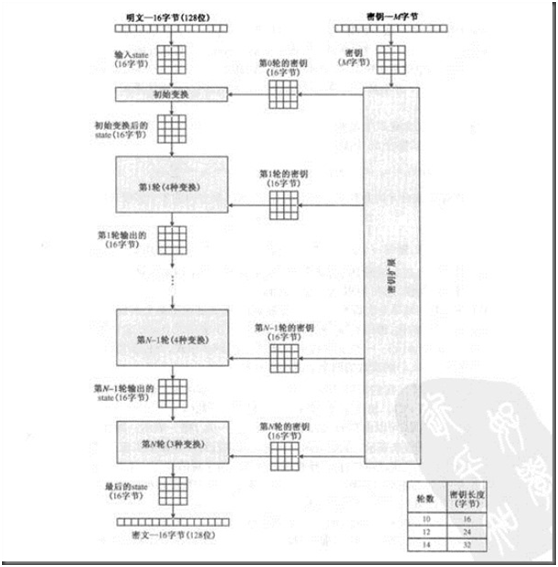2、明文密钥组织方式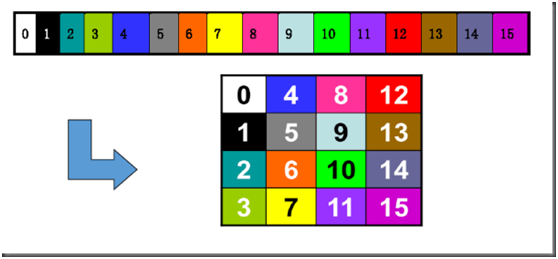3、一些相关的的术语定义和表示

• 状态（State：密码运算的中间结果称为状态。

• State的表示：状态用以字节为基本构成元素的矩阵阵列来表示，该阵列有4行，列数记为Nb Nb=分组长度（bits÷ 32Nb可以取的值为4，对应的分组长度为128bits

• 密码密钥（CipherKey）的表示 Cipher Key类似地用一个4行的矩阵阵列来表示，列数记为NkNk=密钥长度（bits÷32Nk可以取的值为468，对应的密钥长度为128 192 256 bits

4、详细过程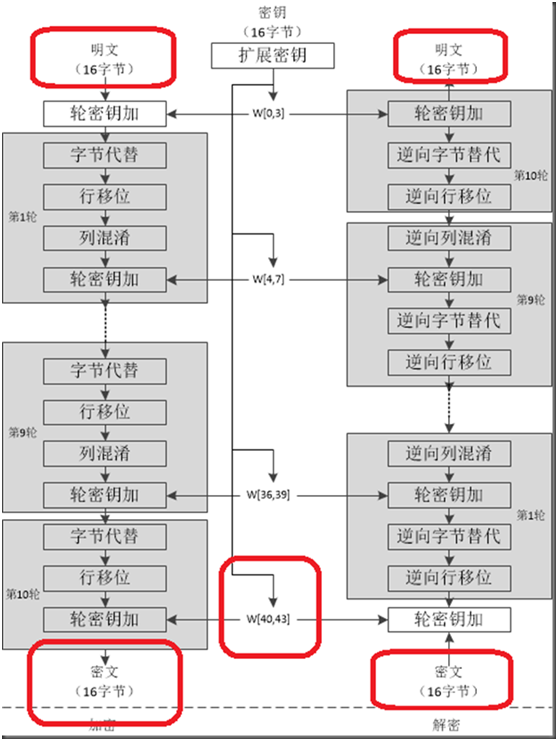1、字节替代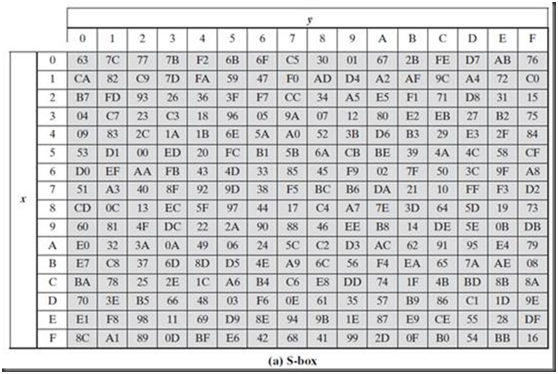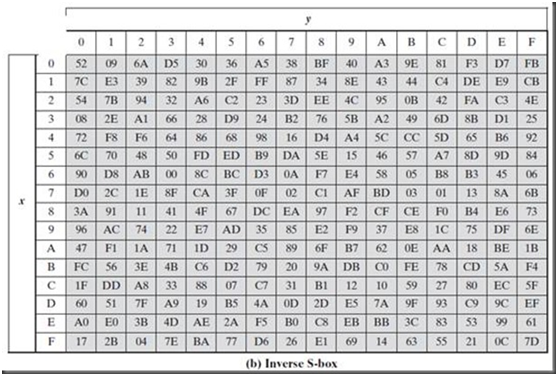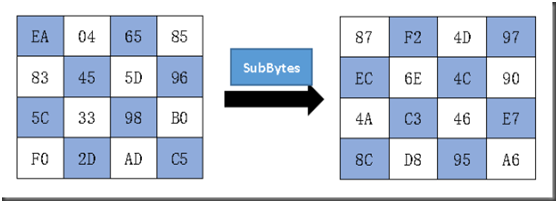2、行移位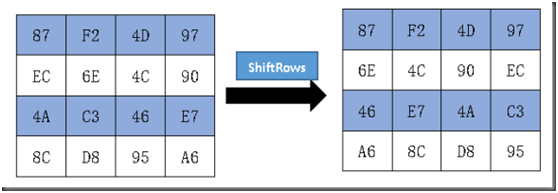3、列混淆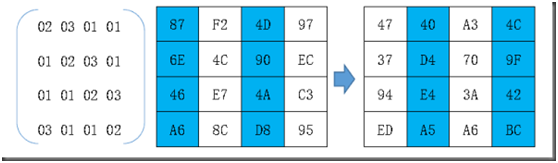47 = (02•87)(03•6E)(01•4A)(01•A6)

02•87 = 02•10000111= 00001110B00011011B =00010101B = 15

03•6E = (0102)•6E = (01•6E)(02•6E)

= 01101110B(11011100B)= 10110010B = B2

01•46 = 46

01•A6 = A6

15B246A6 = 47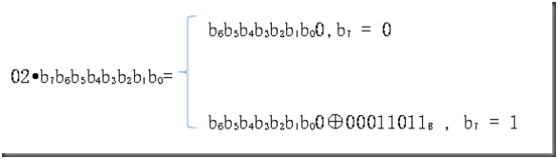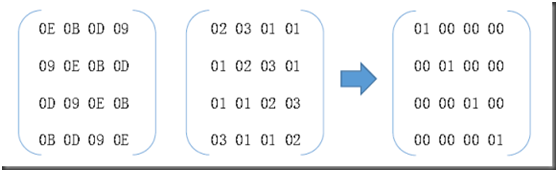4、轮密钥加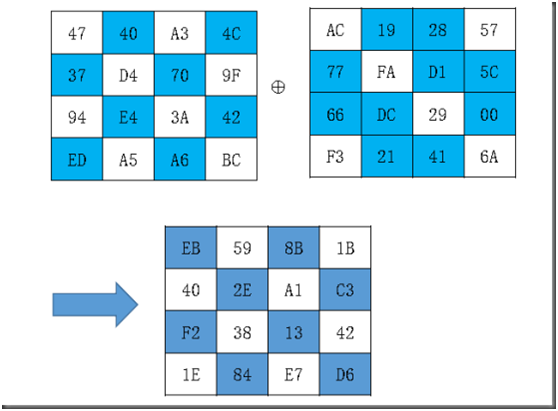ABB = A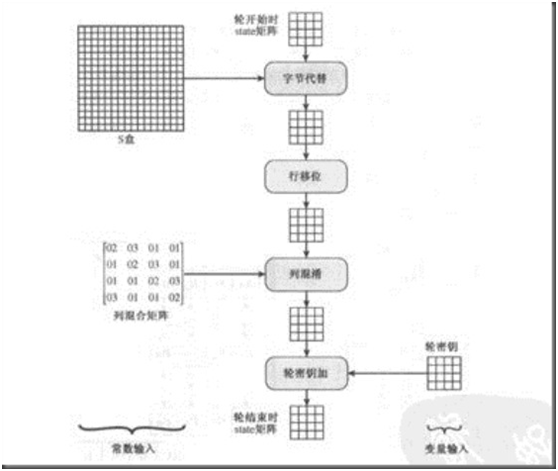5、用伪代码表示的Rijndael加密算法

Rijndael ( State, CipherKey )

{

KeyExpansion ( CipherKey, ExpandedKey );

AddRoundKey ( State, ExpandedKey );

For ( i=1; i<Rnd; i++ )

Round ( State, ExpandedKey+ Nb*i );

FinalRound ( State, ExpandedKey + Nb*Rnd );

}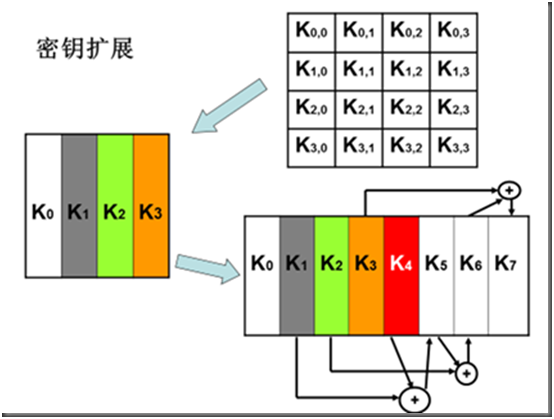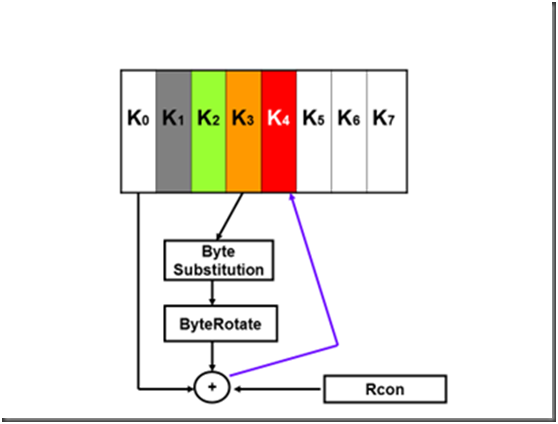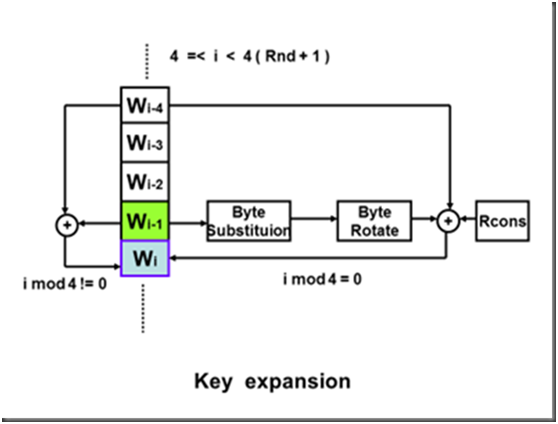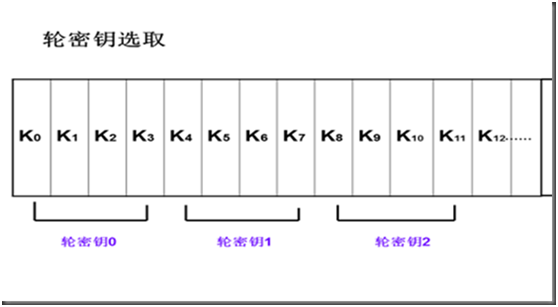KeyExpansion ( byte, Key,word w )

{

word temp；

for (i = 0;i < 4; i++)

w[i] = (key[4*i],key[4*i+1],key[4*i+2], key[4*i+3]);

for (i = 4;i < 44; i++)

{   temp = w[i - 1];

if (i mod 4 = 0) temp =SubWord(RotWord(temp)) + Rcon[i/4];

w[i] = w[i-4] + temp;

}

}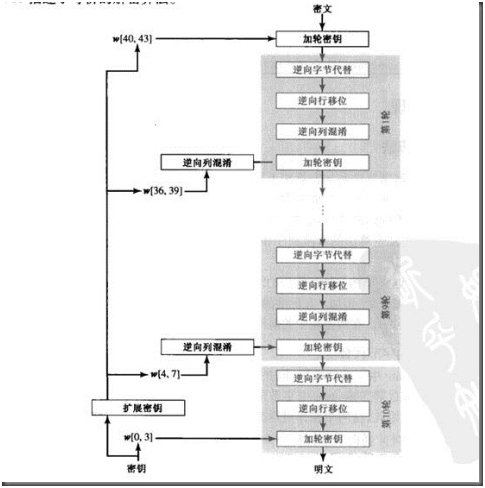Good luck!

Write by Jimmy.li